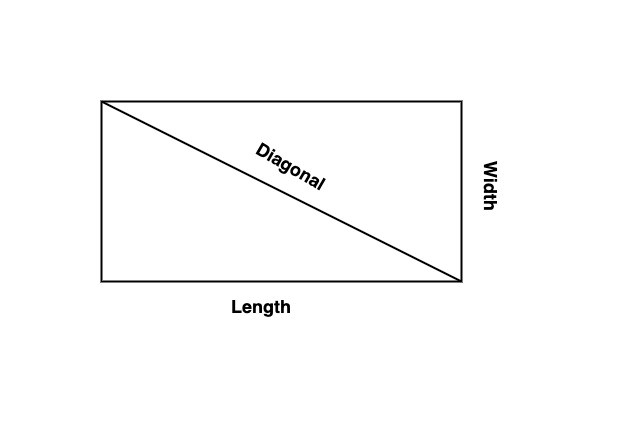# Swift Program to find the area of the rectangle

This tutorial will discuss how to write a Swift program to find the area of the rectangle.

A rectangle is a quadrilateral or a closed two-dimensional shape with four sides. All the angles of a rectangle are equal (90 degrees) and the opposite sides are equal and parallel with each other.The area of the rectangle is known as the total space enclosed inside the boundaries of the rectangle. We can calculate the area of the rectangle by multiplying the length and width.

### Formula for Area of Rectangle

Following is the formula for the area of the rectangle −

Area = Length(L) * Width(W)


Below is a demonstration of the same −

Input

Suppose our given input is −

Length = 10
Width = 5


Output

The desired output would be −

Area = 10 * 5 = 50.
Area = 50


### Algorithm

Following is the algorithm −

• Step 1 − Declare two variables of integer type to store the value of the length and width of the rectangle. Here the value of these variables can be pre-defined or user-defined.

• Step 2 − Declare an Area variable to store the area of the rectangle.

var RectArea = RectLength * RectWidth

• Step 3 − Print the output.

### Example 1

The following program shows how to find the area of the rectangle.

import Foundation
import Glibc

var RectLength : Int = 20
var RectWidth : Int = 10

var RectArea = RectLength * RectWidth

print("Length - ", RectLength)
print("Width - ", RectWidth)
print("So, Area of the rectangle- ", RectArea)


### Output

Length - 20
Width - 10
So, Area of the rectangle- 200


Here, in the above code, we find the area of the rectangle by multiplying the given length and width of the rectangle.

var RectArea = RectLength * RectWidth


That is ReactArea = 20 * 10 = 200. And display the area that is 200

### Example 2

The following program shows how to find the area of the rectangle with user-defined inputs.

import Foundation
import Glibc

// Taking input from the user
print("Please enter the length - ")
print("Please enter the width - ")

// Finding the area of the rectangle
var RectArea = RectLength * RectWidth

print("Entered Length - ", RectLength)
print("Entered Width - ", RectWidth)
print("So, Area of the rectangle- ", RectArea)


### STDIN Input

Please enter the length -
6
3


### Output

Entered Length -  6
Entered Width -  3
So, Area of the rectangle - 18


Here, in the above code, we find the area of the rectangle by multiplying the given length and width of the rectangle.

var RectArea = RectLength * RectW idth


Here we take the value of RectLength and RectWidth from the user using readLine() function and convert the entered value in the integer type using Int() function. So ReactArea = 6 * 3 = 18, And display the result that is 18.

## Area of a rectangle in terms of diagonals

We can also calculate the area of the rectangle with the help of diagonal and width. A rectangle has two diagonals and both are equal.

### Formula for Area of Rectangle using Diagonals

Following is the formula for the area of rectangle in terms of diagonals

Area = Width(√ (Diagonal)2 - (Width)2)


### Example

The following program shows how to find the area of the rectangle using diagonals.

import Foundation
import Glibc

var RectDiagonal = 30.0
var RectWidth = 10.0

// Finding the area of the rectangle in terms of diagonals
var RectArea = RectWidth * (sqrt(pow(RectDiagonal, 2) - pow(RectWidth, 2)))

print("Diagonal - ", RectDiagonal)
print("Width - ", RectWidth)
print("So, Area of the rectangle- ", RectArea)


### Output

Diagonal -  30.0
Width -  10.0
So, Area of the rectangle-  282.842712474619


Here in the above code, we find the area of the rectangle with the help of diagonal and width using the formula −

var RectArea = RectWidth * (sqrt(pow(RectDiagonal, 2) - pow(RectWidth, 2)))


Here we find the square root using sqrt() function and pow() function is used to find the power. So the working of the above code is

ReactArea = 10.0 * (sqrt(pow(30.0, 2) - pow(10.0, 2))) = 10.0 * (sqrt(900 - 100)) = 10.0 * (sqrt(800)) = 10.0 * (28.2842712474619) = 282.842712474619